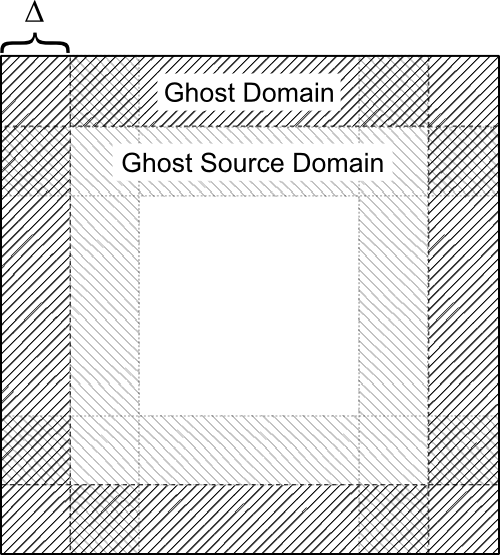# Boundaries

In the previous section we have seen that the `Range` class can be used to define rectangular regions of arbitrary dimension. The `Boundary` class makes use of these regions to define regions of ghost cells and boundary cells.

Ghost cells are parts of the grid that are not part of the inner simulation domain. They are needed for many grid based calculations when the value of a grid cell is updated from the values of the neighbouring cells. On the global simulation boundaries the ghost cells (or boundary cells) have to be filled with values determined by the boundary conditions. On the other hand, in a parallel simulation the ghost cells a one process have to be filled with values from the neighbouring process.

The `Boundary` class makes it easy to obtain the rectangular domains that correspond to the ghost cells on each side of the simulation domain. The image shows the geometry of the simulation domain and the ghost domains.
The `Boundary` class takes two template parameters, the `rank` represents the dimensionality of the grid and `CheckingPolicy` supplies the checking policy.

The class defines three constructors.

```  Boundary();
Boundary(const LimitType &lo, const LimitType &hi, int delta_);
Boundary(DomainType &size_, int delta_);
```

The first of these constructors creates an empty boundary. A `Boundary` created this way needs to be filled with values before it can be used. The second constructor takes the coordinates of the lower and upper corners of the simulation domain, given by `lo` and `high`, and the thickness of the ghost domain given by `delta`. The type `Boundary::LimitType` is simply defined as an `Array`.

```typedef Array<int, rank, CheckingPolicy> LimitType;
```

The third constructor takes a the size of the simulation domain given by `size` and the thickness of the ghost domain given by `delta`. Here the type `Boundary::DomainType` is simply defined as a `Range`.

```typedef Range<int, rank, CheckingPolicy> DomainType;
```

Two inspector methods simply return the simulation domain and the thickness of the ghost domain.

```  const DomainType& getDomain();
int getDelta();
```Figure 1: Schematic diagram of the simulation domain and the ghost cells. The inner simulation domain contains all the cell except the ghost cells.

The following three methods are related to creating the ghost domain and the source region on the neighbouring process form which the ghost domain is filled. Figure 1 shows how these regions are arranged. The ghost domain lies outside the inner domain and surrounds it with a thickness given by `delta`. For parallel simulations the ghost domain must be filled with values from the neighbouring process. The rectangular region that is the source for the ghost domain on the neighbouring process is called ghost source domain.

```  typedef enum {Min, Max} bound;
DomainType getGhostDomain(int dim, bound b);
DomainType getGhostSourceDomain(int dim, bound b);
DomainType getInnerDomain();
```

The method `getGhostDomain()` will return the rectangular range representing the ghost domain on one side of the simulation domain. The arguments `dim` specifies the direction of the boundary and `bound` specifies whether the upper or lower ghost domain should be returned.

The method `getGhostSourceDomain()` will return the rectangular range representing the ghost source domain on one side of the simulation domain. As above, the arguments `dim` specifies the direction of the boundary and `bound` specifies whether the upper or lower ghost source domain should be returned. Note that the lower ghost source domain of one process should be used to fill the upper ghost domain of the neighbouring process.

The following piece of code illustrates the behaviour.

```  Array<int, 3> lo(0,0,0), hi(10, 10, 10);
Boundary<3> boundary(lo, hi, 2);

Range<int, 3> ghost = boundary.getGhostDomain(1,Boundary<3>::Max);
Range<int, 3> source = boundary.getGhostSourceDomain(1,Boundary<3>::Max);
Range<int, 3> inner = boundary.getInnerDomain();
```

A boundary object is initialised with a range from (0,0,0) to (10, 10, 10) and a ghost layer thickness of 2. Then the ghost domain and the ghost source domain of the upper boundary in the y-direction is calculated. After this code has finished the three ranges will have the following values.

```ghost  =  (0, 9, 0) - (10, 10, 10)
source =  (0, 7, 0) - (10, 8, 10)
inner  =  (2, 2, 2) - (8, 8, 8)
```

A method that behaves similar to `getGhostDomain` is `getBoundaryDomain`. However, `getBoundaryDomain` takes grid staggering into account when calculating the boundary domain. This is useful for outer simulation boundaries in which the staggering affects the number of cells in the boundary region.

```  DomainType getBoundaryDomain(int dim, bound b, bool stagger);
```

The arguments have `dim` and `bound` have the same meaning as before. The boolean `stagger` indicated if the grid is staggered by half a grid cell in the direction specified by `dim`.

Instead of calculating just the ghost domain, you can directly create a sub-grid representing the ghost cells.

```  template
SubGrid<GridType, CheckingPolicy> getGhostBoundary(int dim, bound b, GridType &grid);
```

This method takes, in addition to `dim` and `bound`, a grid and returns a `SubGrid` that provides a view on the original grid, restricted to the corresponding boundary domain.

The code for this tutorial can be found here.